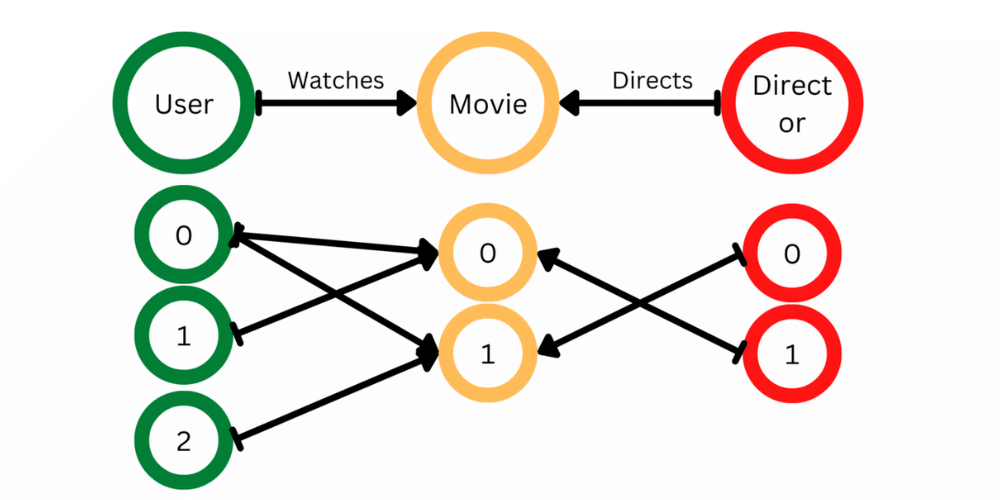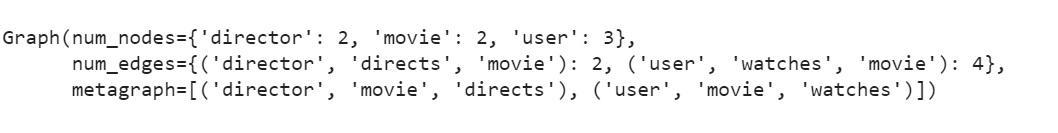Open in App
Not now

# Create Heterogeneous Graph Using dgl in Python

• Difficulty Level : Expert
• Last Updated : 23 Dec, 2022

In this article, we will create Heterogeneous Graphs using dgl (Deep Graph Library) library in Python. Graphs are nothing but collections of Nodes/Vertices and Edges. Also, Edges are nothing but Source nodes to Destination nodes. So all edges can be represented as (U, V) where U and V are nodes.

But in the case of Heterogeneous Graphs, we need to be one step more careful. A heterogeneous graph is a special kind of information network, which contains either multiple types of objects (Nodes) or multiple types of links (Edges).

G = (V, E)

## What is Deep Graph Library (DGL) in Python?

The Deep Graph Library (DGL) is a Python open-source library that helps researchers and scientists quickly build, train, and evaluate GNNs on their datasets. It is Framework Agnostic. Build your models with PyTorch, TensorFlow, or Apache MXNet.

There is just a slight variation when compared to the creation of Homogeneous graphs. In the case of homogeneous graphs, all we need to pass is 2 sets of tensors and it creates edges between the IDs that are being passed. As Homogeneous graphs have only 1 type of node and 1 type of edge.

Here In Heterogeneous graphs, we have multiple types of nodes and multiple types of edges. So the input we pass is a dictionary of key-value pairs for each relationship.

Syntax: { (source_type, edge_type, destination_type)  : (src_node_id Tensor , dest_node_id Tensor ) }Create a Heterogeneous Graph Using dgl in Python

Below is the implementation:

## Python3

 `import` `dgl``import` `torch` `data_dict ``=` `{``    ``(``'user'``, ``'watches'``, ``'movie'``): (torch.tensor([``0``, ``0``, ``1``, ``2``]),``                                   ``torch.tensor([``0``, ``1``, ``0``, ``1``])),``    ``(``'director'``, ``'directs'``, ``'movie'``): (torch.tensor([``0``, ``1``]),``                                       ``torch.tensor([``1``, ``0``]))``}``hetero_graph ``=` `dgl.heterograph(data_dict)``hetero_graph`

Output:Now let’s examine the different types of nodes in the graph we created here.

## Python3

 `hetero_graph.ntypes`

Output:

`['director', 'movie', 'user']`

We can also look at the different types of edges present in the graph at hand.

## Python3

 `hetero_graph.etypes`

Output:

`['directs', 'watches']`

### Benefits of creating a Heterogeneous graph

• If we look at real-world scenarios – most of the problems are of heterogeneous graph type.
• Also, the next benefit is that we get the opportunity to apply different GNN Models to different relationships.
• Also, while negative and positive sampling we get the exact edges, no redundant and ambiguous edges are being selected.
• Here a good example is a User – (watches) -> Movie. This watches relationship can be between a user and a movie only. You cannot have Movie- (watches) -> Movie (or) User – (watches) -> User relationships in the case of heterogeneous graphs, as we know the type of the node.

My Personal Notes arrow_drop_up